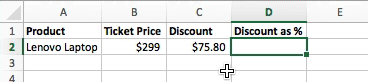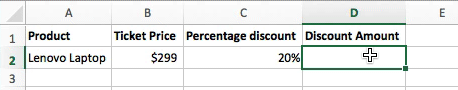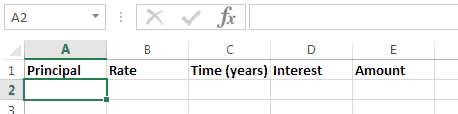### Excel formula

#### Discount as percentage | # gomaths.net/4784    User submitted, thanks to Simon Job

Calculate discount as a percentage from sale price and discount amount.

`=C2/B2`#### Percentage discount | # gomaths.net/4785    User submitted, thanks to Simon Job

Calculate a percentage discount.

`=B2-C2*B2`#### Simple interest | # gomaths.net/4222    User submitted, thanks to Simon Job

Calculate simple interest.

`=A2*B2*C2` (calculate simple interest)

`=A2+D2` (amount)

where `A2` is the Principal
`B2` is the interest rate as a percentage
`C2` is the time in years